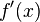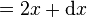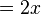# Infinitesimal

An infinitesimal is a quantity that is so small that it cannot be seen or measured. In mathematics, it is a non-zero quantity that approaches zero as a limit. When used as an adjective in the vernacular, infinitesimal means extremely small. In everyday life, an infinitesimal object is one that is smaller than any possible measure, whether we measure size, time, chemical concentration, or other property.

Before the nineteenth century, none of the mathematical concepts as we know them today were formally defined, but many of these concepts were already there. The founders of calculus—Leibniz, Newton, Euler, Lagrange, the Bernoullis and many others—used infinitesimals in the way shown below and achieved essentially correct results, although no formal definition was available. (Likewise, there was no formal definition of real numbers at the time).

## History of the infinitesimal

The first mathematician to make use of infinitesimals was Archimedes (around 250 B.C.E.). The Archimedean property is the property of an ordered algebraic structure having no nonzero infinitesimals.

In India, from the twelfth to the sixteenth century, infinitesimals were discovered for use with differential calculus by Indian mathematician Bhaskara and various Keralese mathematicians.

When Newton and Leibniz developed calculus, they made use of infinitesimals. A typical argument might go as follows:

To find the derivative f′(x) of the function f(x) = x2, let dx be an infinitesimal. Then,$f'(x)\,$$=\frac{f(x + \mathrm dx) - f(x)}{\mathrm dx}\,$$=\frac{x^2 + 2x \cdot \mathrm dx + \mathrm dx^2 -x^2}{\mathrm dx}\,$$=2x + \mathrm dx\,$$=2x\,$
since dx is infinitely small.

This argument, while intuitively appealing, and producing the correct result, is not mathematically rigorous. The use of infinitesimals was attacked as incorrect by Bishop Berkeley in his work The Analyst. The fundamental problem is that dx is first treated as non-zero (because we divide by it), but later discarded as if it were zero.

When we consider numbers, the naive definition is clearly flawed: an infinitesimal is a number whose modulus is less than any non-zero positive number. Considering positive numbers, the only way for a number to be less than all numbers would be to be the least positive number. If h is such a number, then what is h/2? Or, if h is indivisible, is it still a number? Also, intuitively, one would require the reciprocal of an infinitesimal to be infinitely large (in modulus) or unlimited. That step should yield the "largest" number, but clearly there is no "last" biggest number.

It was not until the second half of the nineteenth century that the calculus was given a formal mathematical foundation by Karl Weierstrass and others using the notion of a limit. In the twentieth century, it was found that infinitesimals could, after all, be treated rigorously. Neither formulation is wrong, and both give the same results if used correctly.

## Modern uses of infinitesimals

Infinitesimal is necessarily a relative concept. If epsilon is infinitesimal with respect to a class of numbers, it means that epsilon cannot belong to that class. This is the crucial point: infinitesimal must necessarily mean infinitesimal with respect to some other type of numbers.

### The path to formalization

Proving or disproving the existence of infinitesimals of the kind used in nonstandard analysis depends on the model and which collection of axioms are used. We consider here systems where infinitesimals can be shown to exist.

In 1936 Maltsev proved the compactness theorem. This theorem is fundamental for the existence of infinitesimals as it proves that it is possible to formalize them. A consequence of this theorem is that if there is a number system in which it is true that for any positive integer n there is a positive number x such that 0< x < 1/n, then there exists an extension of that number system in which it is true that there exists a positive number x such that for any positive integer n we have 0 < x < 1/n. The possibility to switch “for any" and "there exists" is crucial. The first statement is true in the real numbers as given in ZFC set theory: for any positive integer n it is possible to find a real number between 1/n and zero, only this real number will depend on n. Here, one chooses n first, then one finds the corresponding x. In the second expression, the statement says that there is an ‘x’’ (at least one), chosen first, which is between 0 and 1/n for any n. In this case x is infinitesimal. This is not true in the real numbers (R) given by ZFC. Nonetheless, the theorem proves that there is a model (a number system) in which this will be true. The question is: what is this model? What are its properties? Is there only one such model?

There are in fact many ways to construct such a one-dimensional linearly ordered set of numbers, but fundamentally, there are two different approaches:

1) Extend the number system so that it contains more numbers than the real numbers.
2) Extend the axioms (or extend the language) so that the distinction between the infinitesimals and non-infinitesimals can be made in the real numbers.

In 1960, Abraham Robinson provided an answer following the first approach. The extended set is called the hyperreals and contains numbers less in absolute value than any positive real number. The method may be considered relatively complex but it does prove that infinitesimals exist in the universe of ZFC set theory. The real numbers are called standard numbers and the new non-real hyperreals are called nonstandard.

In 1977 Edward Nelson provided an answer following the second approach. The extended axioms are IST, which stands either for Internal Set Theory or for the initials of the three extra axioms: Idealization, Standardization, Transfer. In this system we consider that the language is extended in such a way that we can express facts about infinitesimals. The real numbers are either standard or nonstandard. An infinitesimal is a nonstandard real number which is less, in absolute value, than any positive standard real number.

In 2006 Karel Hrbacek developed an extension of Nelson's approach in which the real numbers are stratified in (infinitely) many levels, i.e, in the coarsest level there are no infinitesimals nor unlimited numbers. Infinitesimals are in a finer level and there are also infinitesimals with respect to this new level and so on.

All of these approaches are mathematically rigorous.

This allows for a definition of infinitesimals which refers to these approaches:

### A definition

An infinitesimal number is a nonstandard number whose modulus is less than any nonzero positive standard number.

What standard and nonstandard refer to depends on the chosen context.

Alternatively, we can have synthetic differential geometry or smooth infinitesimal analysis with its roots in category theory. This approach departs dramatically from the classical logic used in conventional mathematics by denying the law of excluded middle—i.e., not (ab) does not have to mean a = b. A nilsquare or nilpotent infinitesimal can then be defined. This is a number x where x2 = 0 is true, but x = 0 need not be true at the same time. With an infinitesimal such as this, algebraic proofs using infinitesimals are quite rigorous, including the one given above.